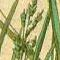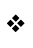Back to List

Plain
Coloured
Gaudy

This code
in plain text

### Browsing parserm.h

TryNumber (lines 3432-3484)

```3432  !  TryNumber is the only routine which really does any character-level
3433  !  parsing, since that's normally left to the Z-machine.
3434  !  It takes word number "wordnum" and tries to parse it as an (unsigned)
3435  !  decimal number, returning
3436  !
3437  !  -1000                if it is not a number
3438  !  the number           if it has between 1 and 4 digits
3439  !  10000                if it has 5 or more digits.
3440  !
3441  !  (The danger of allowing 5 digits is that Z-machine integers are only
3442  !  16 bits long, and anyway this isn't meant to be perfect.)
3443  !
3444  !  Using NumberWord, it also catches "one" up to "twenty".
3445  !
3446  !  Note that a game can provide a ParseNumber routine which takes priority,
3447  !  to enable parsing of odder numbers ("x45y12", say).
3448  ! ----------------------------------------------------------------------------
3449
3450  [ TryNumber wordnum   i j c num len mul tot d digit;
3451
3452     i=wn; wn=wordnum; j=NextWord(); wn=i;
3453     j=NumberWord(j); if (j>=1) return j;
3454
3455     i=wordnum*4+1; j=parse->i; num=j+buffer; len=parse->(i-1);
3456
3458
3459     if (len>=4) mul=1000;
3460     if (len==3) mul=100;
3461     if (len==2) mul=10;
3462     if (len==1) mul=1;
3463
3464     tot=0; c=0; len=len-1;
3465
3466     for (c=0:c<=len:c++)
3467     {   digit=num->c;
3468         if (digit=='0') { d=0; jump digok; }
3469         if (digit=='1') { d=1; jump digok; }
3470         if (digit=='2') { d=2; jump digok; }
3471         if (digit=='3') { d=3; jump digok; }
3472         if (digit=='4') { d=4; jump digok; }
3473         if (digit=='5') { d=5; jump digok; }
3474         if (digit=='6') { d=6; jump digok; }
3475         if (digit=='7') { d=7; jump digok; }
3476         if (digit=='8') { d=8; jump digok; }
3477         if (digit=='9') { d=9; jump digok; }
3478         return -1000;
3479       .digok;
3480         tot=tot+mul*d; mul=mul/10;
3481     }
3482     if (len>3) tot=10000;